# Chapter 6 Pseudocode algorithms using sequence selection and

• Slides: 14Chapter 6 Pseudocode algorithms using sequence, selection and repetitionObjectives • To develop solution algorithms to eight typical programming problems using sequence, selection and repetition constructs6. 1 Eight solution algorithmsEight solution algorithms • Each programming problem will be defined, the control structures required will be determined and a solution algorithm will be devised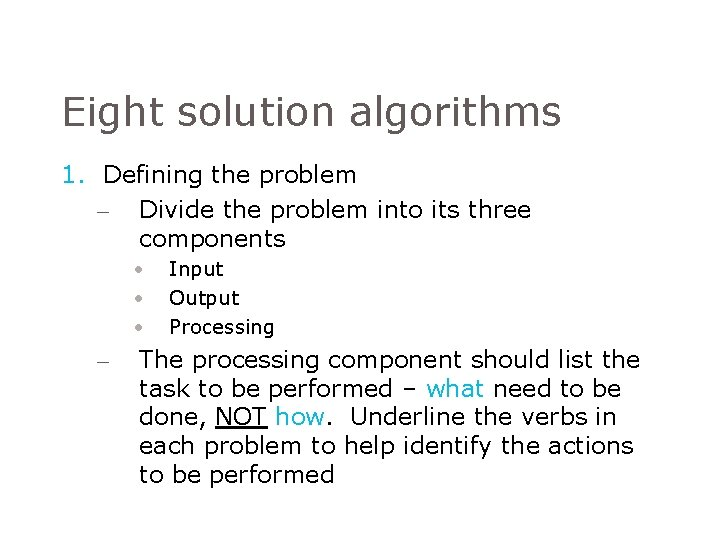Eight solution algorithms 1. Defining the problem – Divide the problem into its three components • • • – Input Output Processing The processing component should list the task to be performed – what need to be done, NOT how. Underline the verbs in each problem to help identify the actions to be performedEight solution algorithms 2. The control structures required – Once the problem has been defined, write down the control structures (sequence, selection and repetition) that may be needed, as well as any extra variables that the solution may require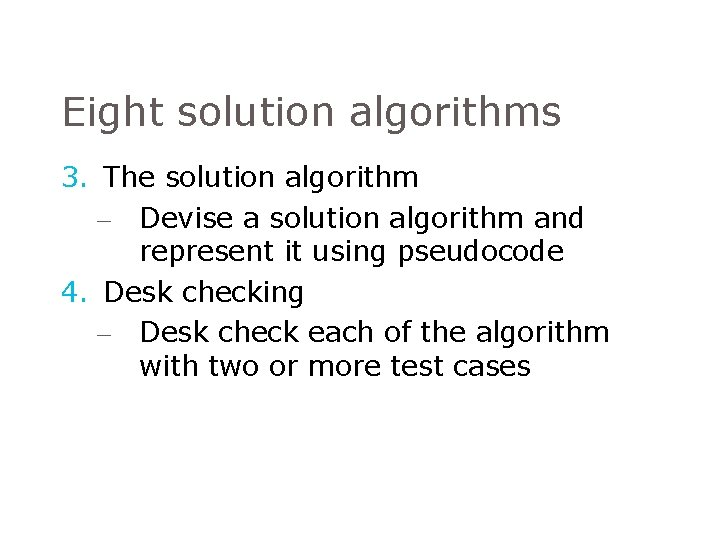Eight solution algorithms 3. The solution algorithm – Devise a solution algorithm and represent it using pseudocode 4. Desk checking – Desk check each of the algorithm with two or more test cases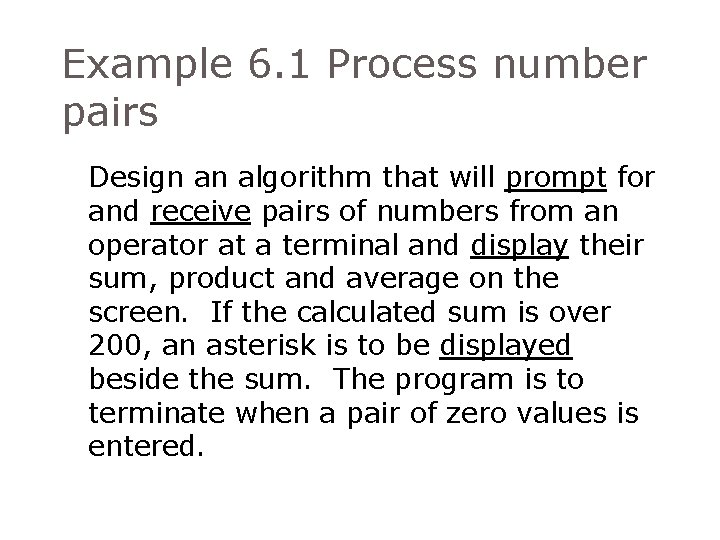Example 6. 1 Process number pairs Design an algorithm that will prompt for and receive pairs of numbers from an operator at a terminal and display their sum, product and average on the screen. If the calculated sum is over 200, an asterisk is to be displayed beside the sum. The program is to terminate when a pair of zero values is entered.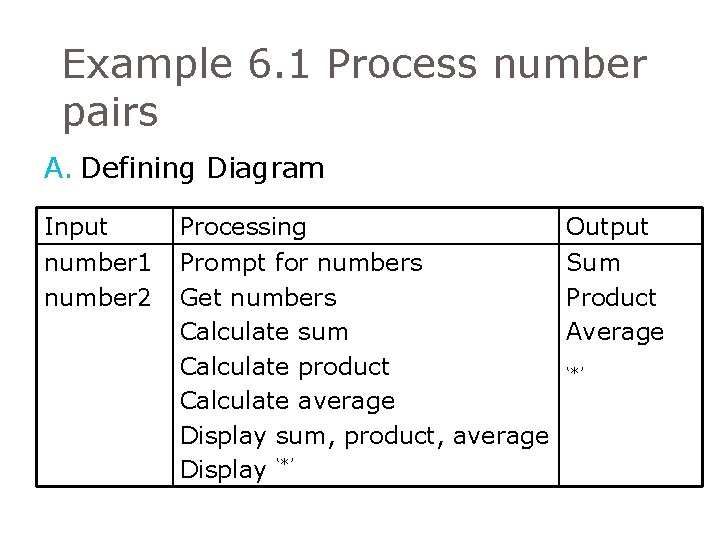Example 6. 1 Process number pairs A. Defining Diagram Input number 1 number 2 Processing Prompt for numbers Get numbers Calculate sum Calculate product Calculate average Display sum, product, average Display ‘*’ Output Sum Product Average ‘*’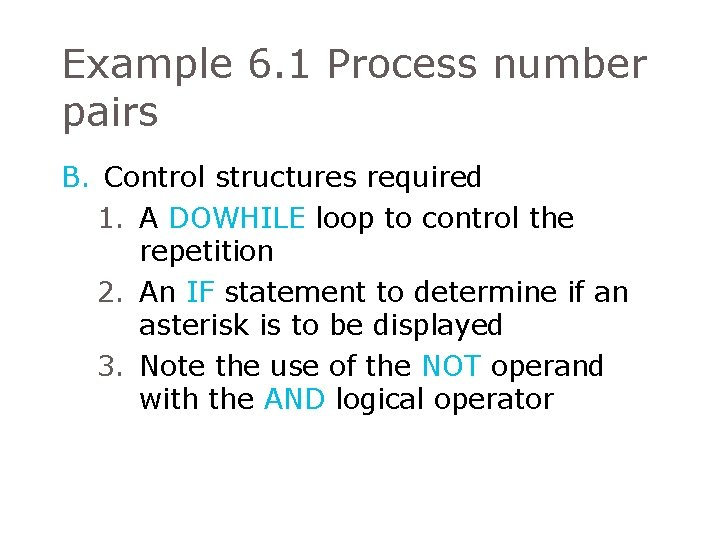Example 6. 1 Process number pairs B. Control structures required 1. A DOWHILE loop to control the repetition 2. An IF statement to determine if an asterisk is to be displayed 3. Note the use of the NOT operand with the AND logical operator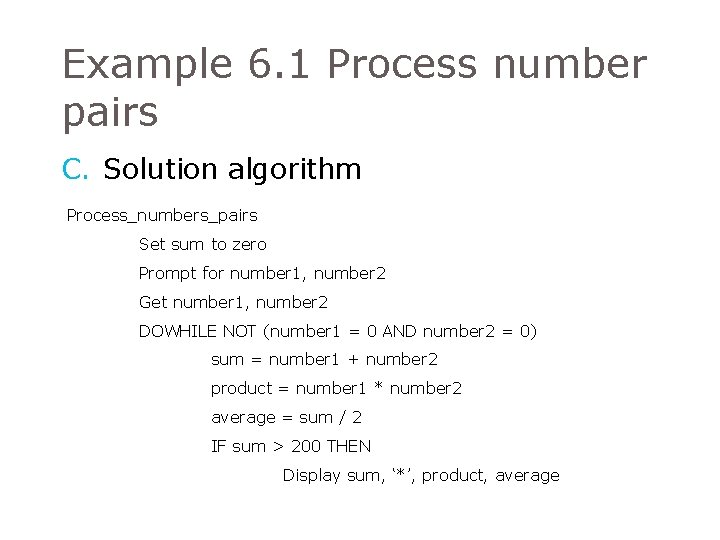Example 6. 1 Process number pairs C. Solution algorithm Process_numbers_pairs Set sum to zero Prompt for number 1, number 2 Get number 1, number 2 DOWHILE NOT (number 1 = 0 AND number 2 = 0) sum = number 1 + number 2 product = number 1 * number 2 average = sum / 2 IF sum > 200 THEN Display sum, ‘*’, product, averageExample 6. 1 Process number pairs ELSE Display sum, product, average ENDIF Prompt for number 1, number 2 Get number 1, number 2 ENDDO END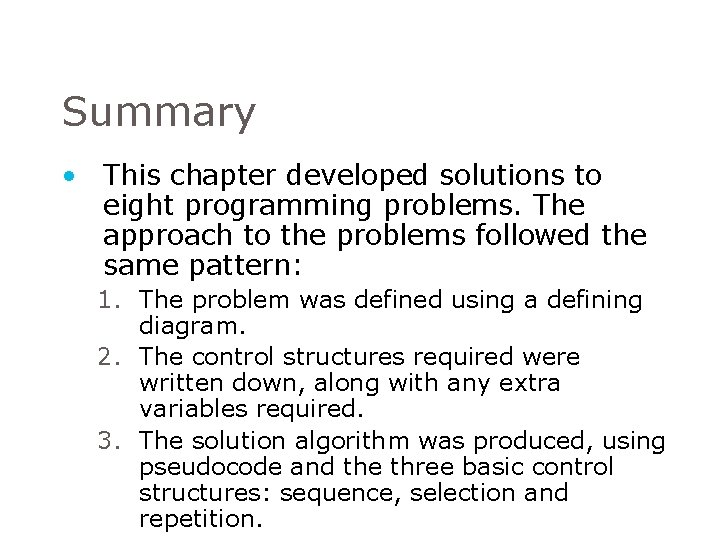Summary • This chapter developed solutions to eight programming problems. The approach to the problems followed the same pattern: 1. The problem was defined using a defining diagram. 2. The control structures required were written down, along with any extra variables required. 3. The solution algorithm was produced, using pseudocode and the three basic control structures: sequence, selection and repetition.Summary • It was noted that the solution algorithms followed the same basic pattern, although the statements within the pattern were different Process_sequential_file Initial processing Read first record DOWHILE more records exist Process this record Read next record ENDDO Final processing END